Creates a tmap-element that draws coordinate grid lines. It serves as a layer that can be drawn anywhere between other layers. By default, tm_grid() draws horizontal and vertical lines according to the coordinate system of the (master) shape object. Latitude and longitude graticules are drawn with tm_graticules().

## Usage

tm_graticules(
x = NA,
y = NA,
n.x = NA,
n.y = NA,
crs = 4326,
labels.format = list(suffix = intToUtf8(176)),
labels.cardinal = TRUE,
...
)

tm_grid(
x = NA,
y = NA,
n.x = NA,
n.y = NA,
crs = NA,
col = NA,
lwd = 1,
alpha = NA,
labels.show = TRUE,
labels.pos = c("left", "bottom"),
labels.size = 0.6,
labels.col = NA,
labels.rot = c(0, 0),
labels.format = list(big.mark = ","),
labels.cardinal = FALSE,
labels.margin.x = 0,
labels.margin.y = 0,
labels.space.x = NA,
labels.space.y = NA,
labels.inside.frame = FALSE,
ticks = labels.show & !labels.inside.frame,
lines = TRUE,
ndiscr = 100,
zindex = NA,
group = NA,
group.control = "none",
...
)

## Arguments

x

X coordinates for vertical grid lines. If NA, it is specified with a pretty scale and n.x.

y

Y coordinates for horizontal grid lines. If NA, it is specified with a pretty scale and n.y.

n.x

Preferred number of grid lines for the x axis. For the labels, a pretty() sequence is used, so the number of actual labels may be different than n.x.

n.y

Preferred number of grid lines for the y axis. For the labels, a pretty() sequence is used, so the number of actual labels may be different than n.y.

crs

Projection character. If specified, the grid lines are projected accordingly. Many world maps are projected, but still have latitude longitude (EPSG 4326) grid lines.

labels.format

List of formatting options for the grid labels. Parameters are:

fun

Function to specify the labels. It should take a numeric vector, and should return a character vector of the same size. By default it is not specified. If specified, the list items scientific, format, and digits (see below) are not used.

scientific

Should the labels be formatted scientifically? If so, square brackets are used, and the format of the numbers is "g". Otherwise, format="f", and text.separator, text.less.than, and text.or.more are used. Also, the numbers are automatically rounded to millions or billions if applicable.

format

By default, "f", i.e. the standard notation xxx.xxx, is used. If scientific=TRUE then "g", which means that numbers are formatted scientifically, i.e. n.dddE+nn if needed to save space.

digits

Number of digits after the decimal point if format="f", and the number of significant digits otherwise.

...

Other arguments passed on to formatC()

labels.cardinal

Add the four cardinal directions (N, E, S, W) to the labels, instead of using negative coordinates for west and south (so it assumes that the coordinates are positive in the north-east direction).

...

Arguments passed on to tm_grid()

col

Color of the grid lines.

lwd

Line width of the grid lines

alpha

Alpha transparency of the grid lines. Number between 0 and 1. By default, the alpha transparency of col is taken.

labels.show

Show tick labels. Either one value for both x and y axis, or a vector two: the first for x and latter for y.

labels.pos

position of the labels. Vector of two: the horizontal ("left" or "right") and the vertical ("top" or "bottom") position.

labels.size

Font size of the tick labels

labels.col

Font color of the tick labels

labels.rot

Rotation angles of the labels. Vector of two values: the first is the rotation angle (in degrees) of the tick labels on the x axis and the second is the rotation angle of the tick labels on the y axis. Only 0, 90, 180, and 270 are valid values.

labels.margin.x

Margin between tick labels of x axis and the frame. Note that when labels.inside.frame == FALSE and ticks == TRUE, the ticks will be adjusted accordingly.

labels.margin.y

Margin between tick labels of y axis and the frame. Note that when labels.inside.frame == FALSE and ticks == TRUE, the ticks will be adjusted accordingly.

labels.space.x

Space that is used for the labels and ticks for the x-axis when labels.inside.frame == FALSE. By default, it is determined automatically using the widths and heights of the tick labels. The unit of this parameter is text line height.

labels.space.y

Space that is used for the labels and ticks for the y-axis when labels.inside.frame == FALSE. By default, it is determined automatically using the widths and heights of the tick labels. The unit of this parameter is text line height.

labels.inside.frame

Show labels inside the frame? By default FALSE.

ticks

If labels.inside.frame = FALSE, should ticks can be drawn between the labels and the frame? Either one value for both x and y axis, or a vector two: the first for x and latter for y.

lines

If labels.inside.frame = FALSE, should grid lines can be drawn?

ndiscr

Number of points to discretize a parallel or meridian (only applicable for curved grid lines)

zindex

zindex of the pane in view mode. By default, it is set to the layer number plus 400. By default, the tmap layers will therefore be placed in the custom panes "tmap401", "tmap402", etc., except for the base tile layers, which are placed in the standard "tile". This parameter determines both the name of the pane and the z-index, which determines the pane order from bottom to top. For instance, if zindex is set to 500, the pane will be named "tmap500".

group

Name of the group to which this layer belongs. This is only relevant in view mode, where layer groups can be switched (see group.control)

group.control

In view mode, the group control determines how layer groups can be switched on and off. Options: "radio" for radio buttons (meaning only one group can be shown), "check" for check boxes (so multiple groups can be shown), and "none" for no control (the group cannot be (de)selected).

## Examples

current.mode <- tmap_mode("plot")
#> tmap mode set to 'plot'

tm_shape(NLD_muni) +
tm_polygons() +
tm_grid()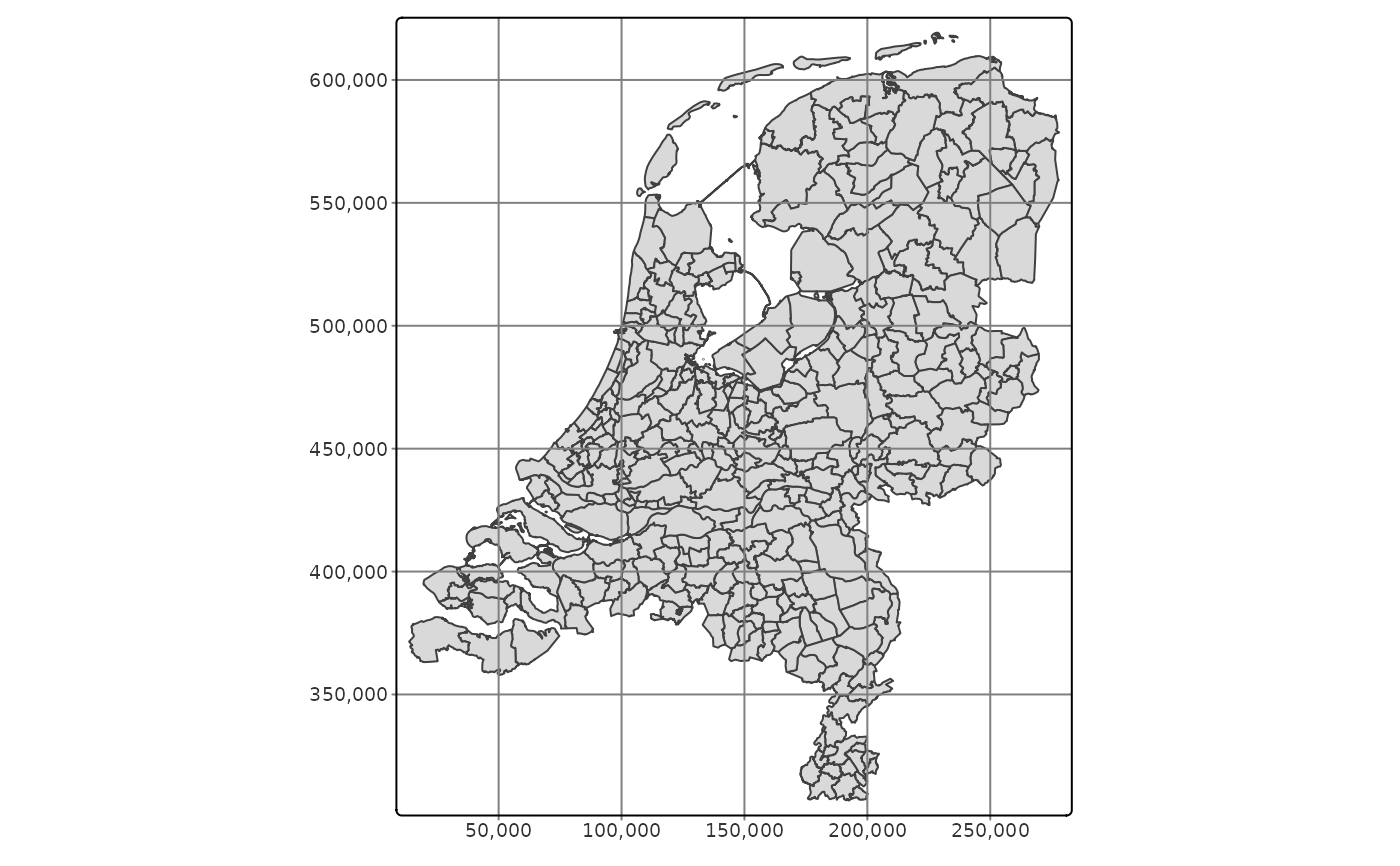tm_shape(NLD_muni) +
tm_polygons() +
tm_grid(crs = 4326)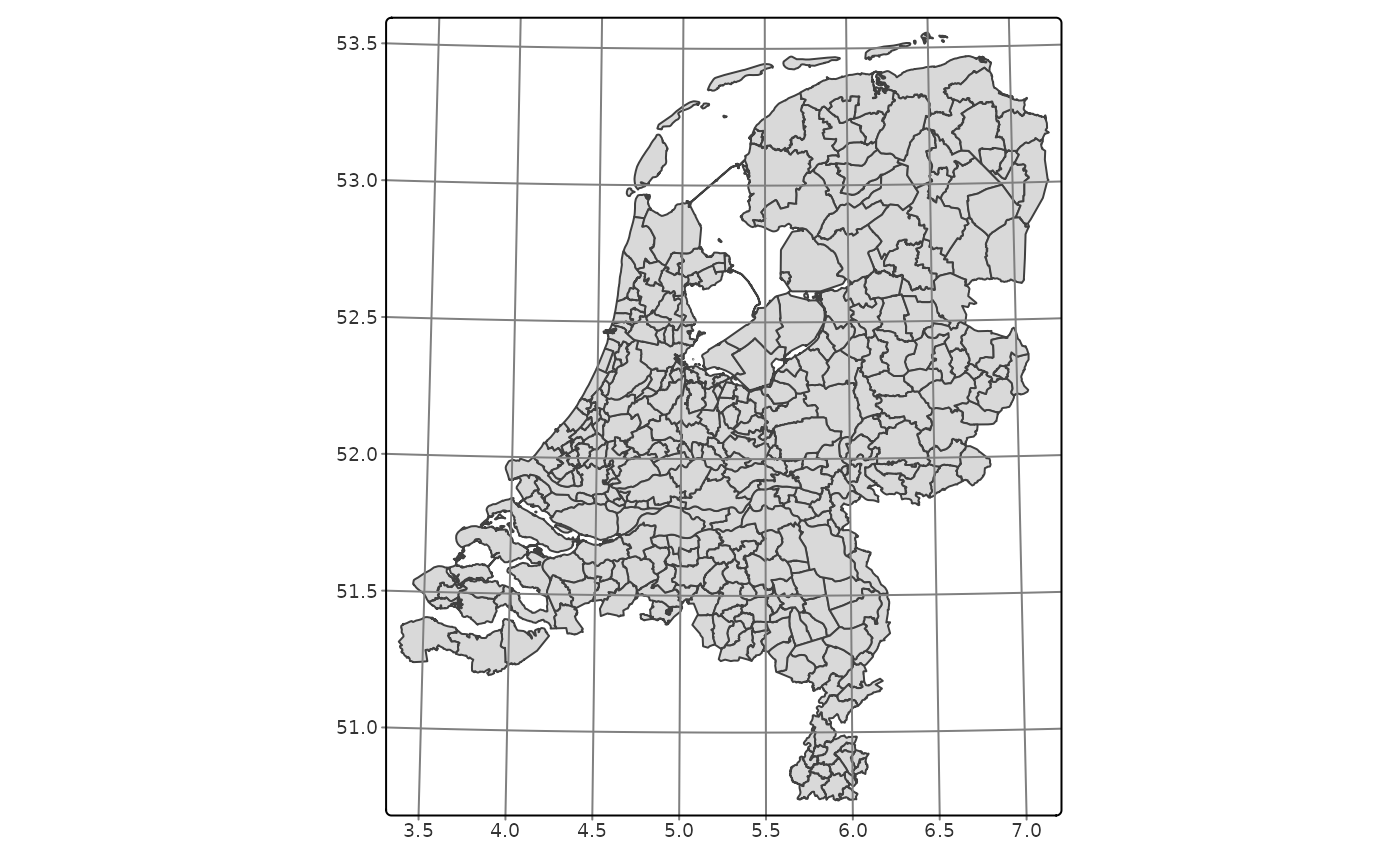tm_shape(NLD_muni) +
tm_polygons() +
tm_grid(crs = 3035, labels.inside.frame = TRUE)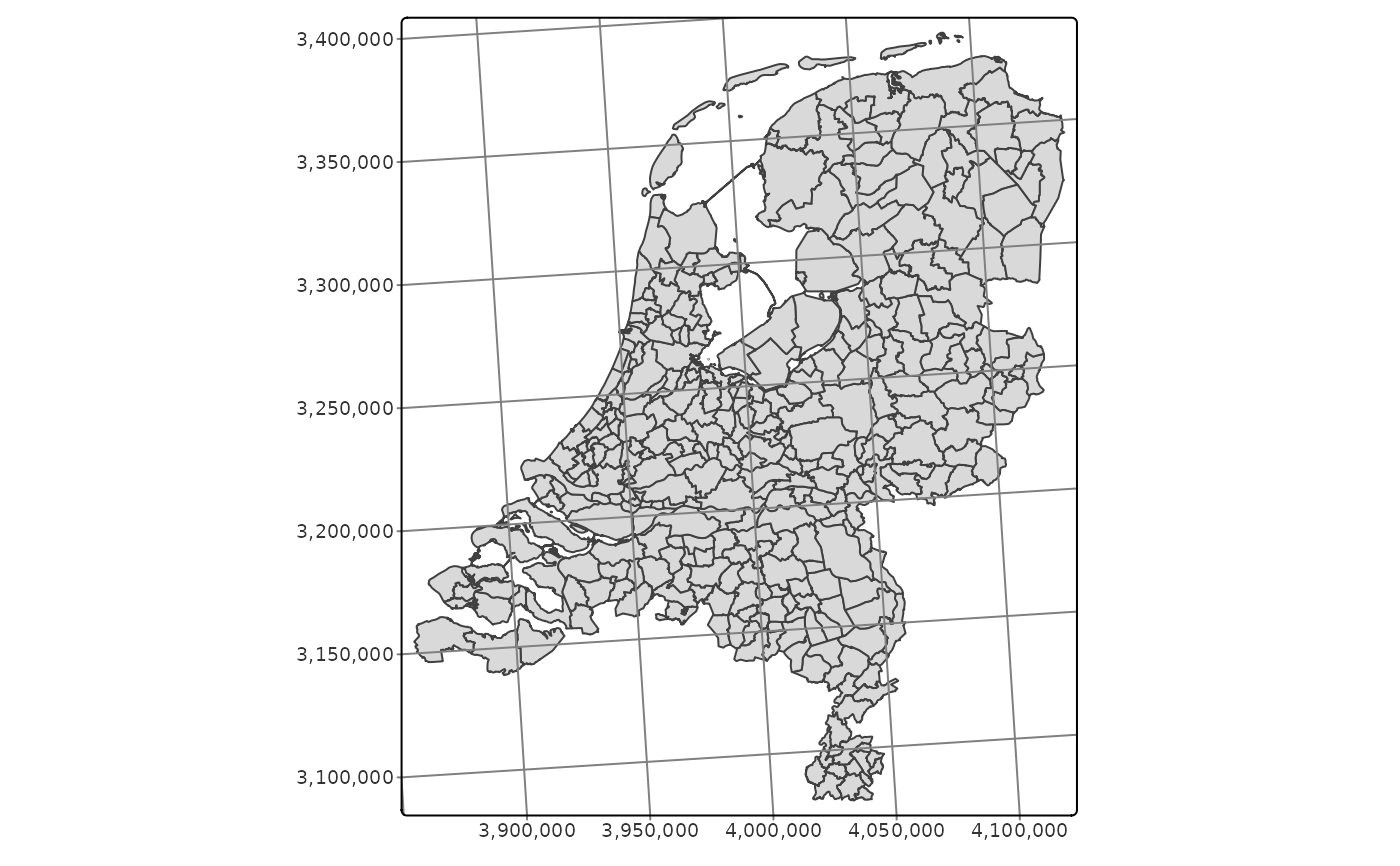tm_shape(World) +
tm_polygons() +
tm_facets(by = "continent") +
tm_grid(labels.inside.frame = TRUE)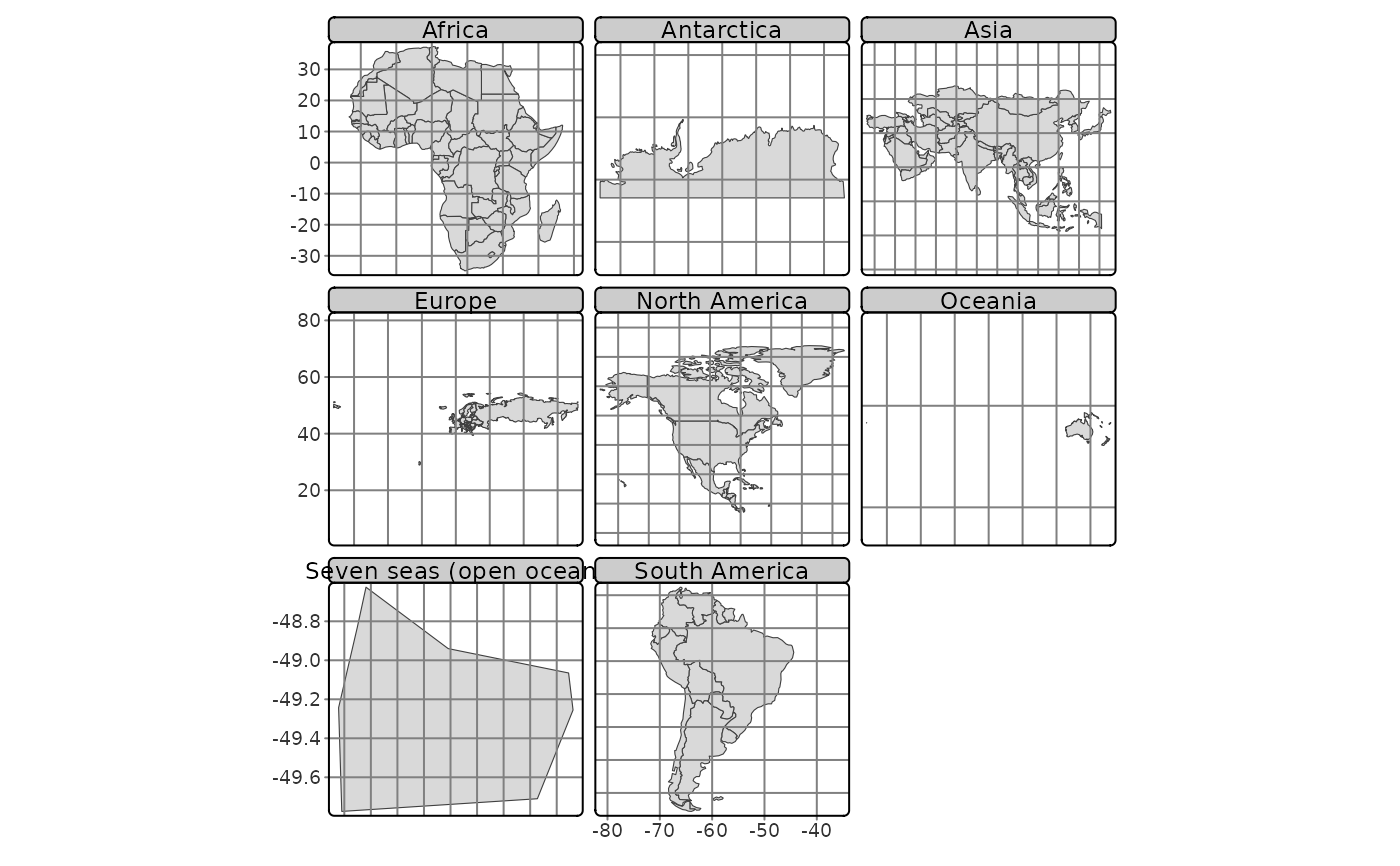tm_shape(NLD_muni) +
tm_polygons() +
tm_graticules()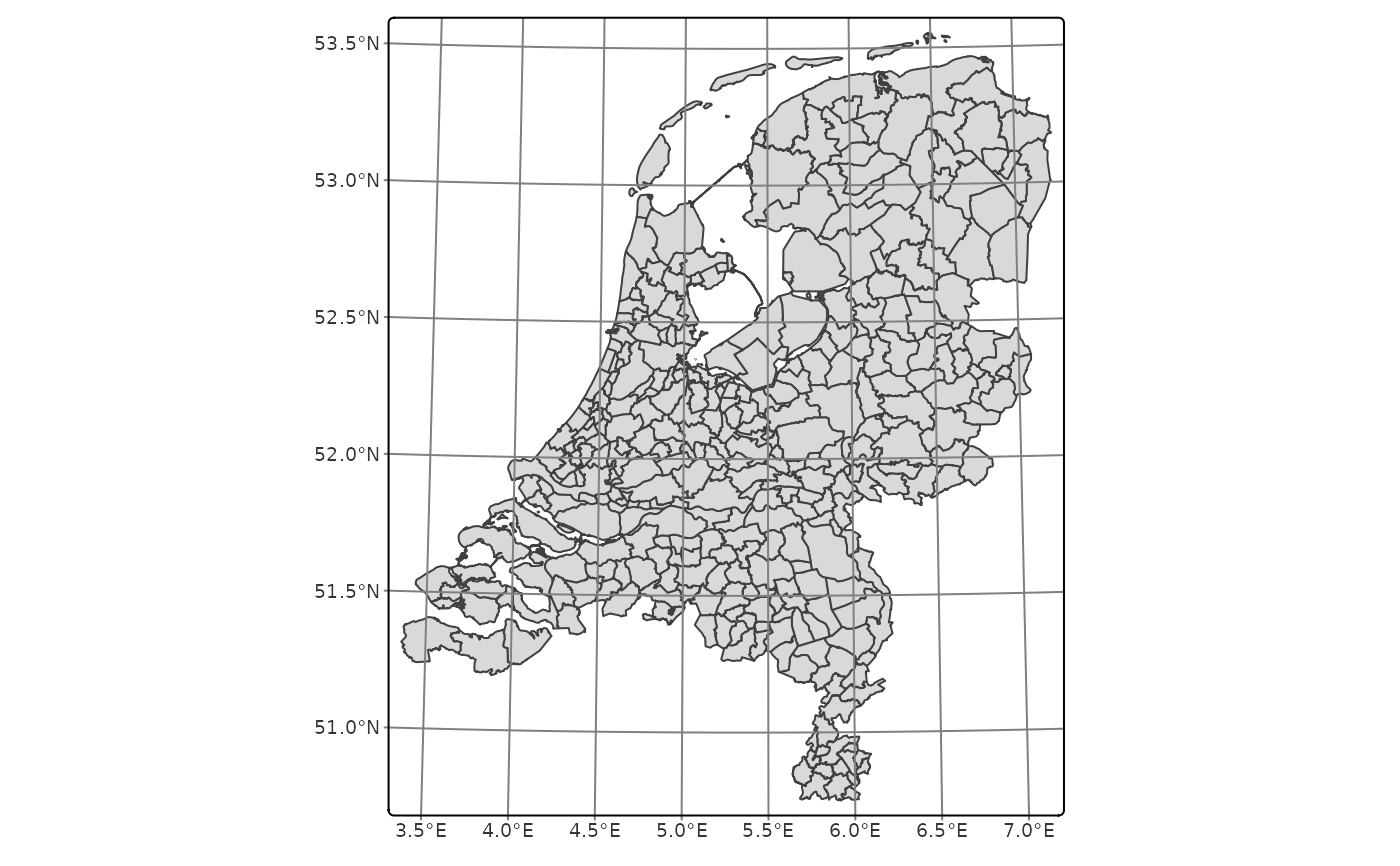tm_shape(NLD_muni) +
tm_polygons() +
tm_graticules(labels.pos = c("right", "top"))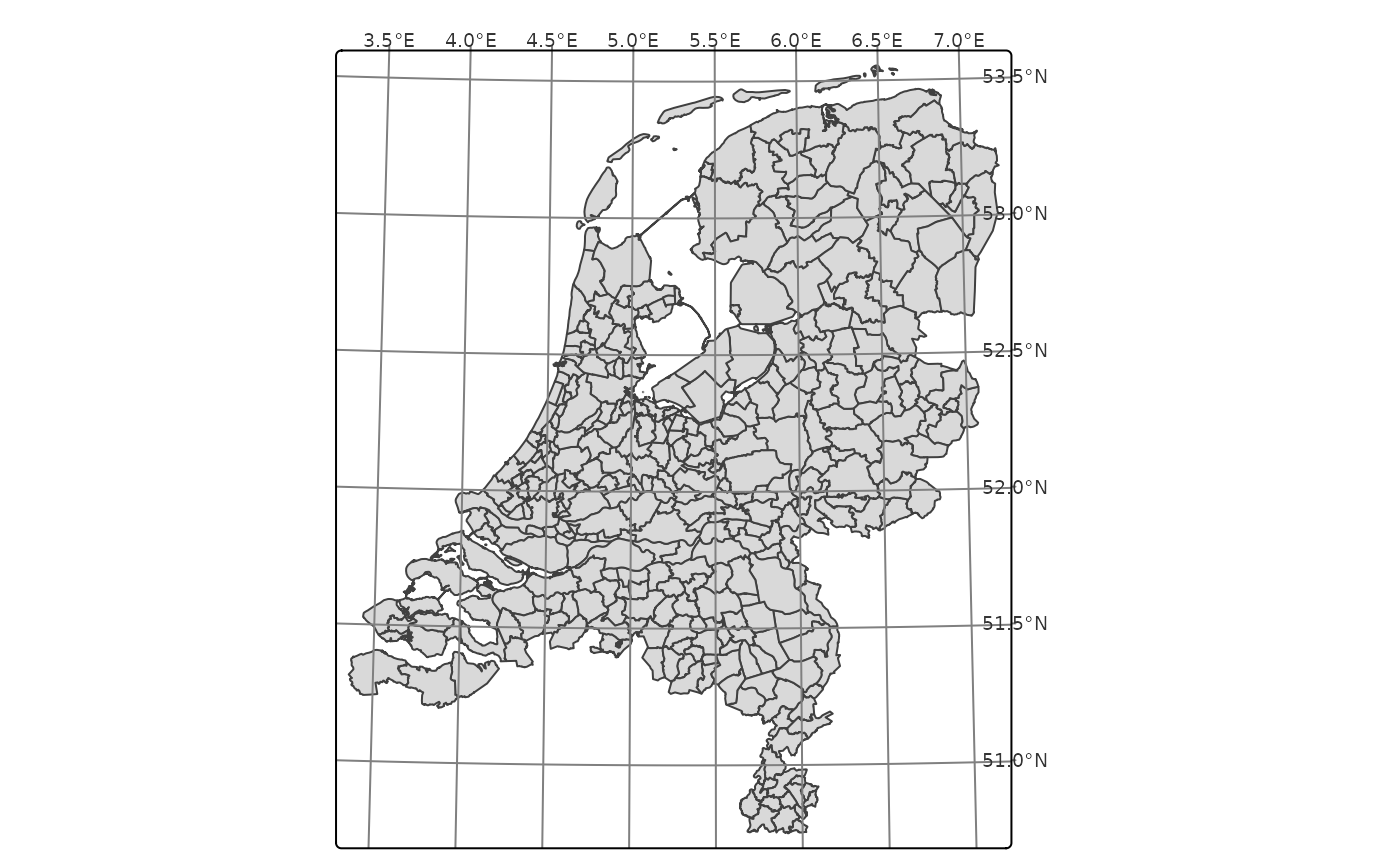data(NLD_muni, World)

tmap_arrange(
qtm(NLD_muni, borders = NULL) + tm_grid(),
qtm(NLD_muni, borders = NULL) + tm_graticules()
)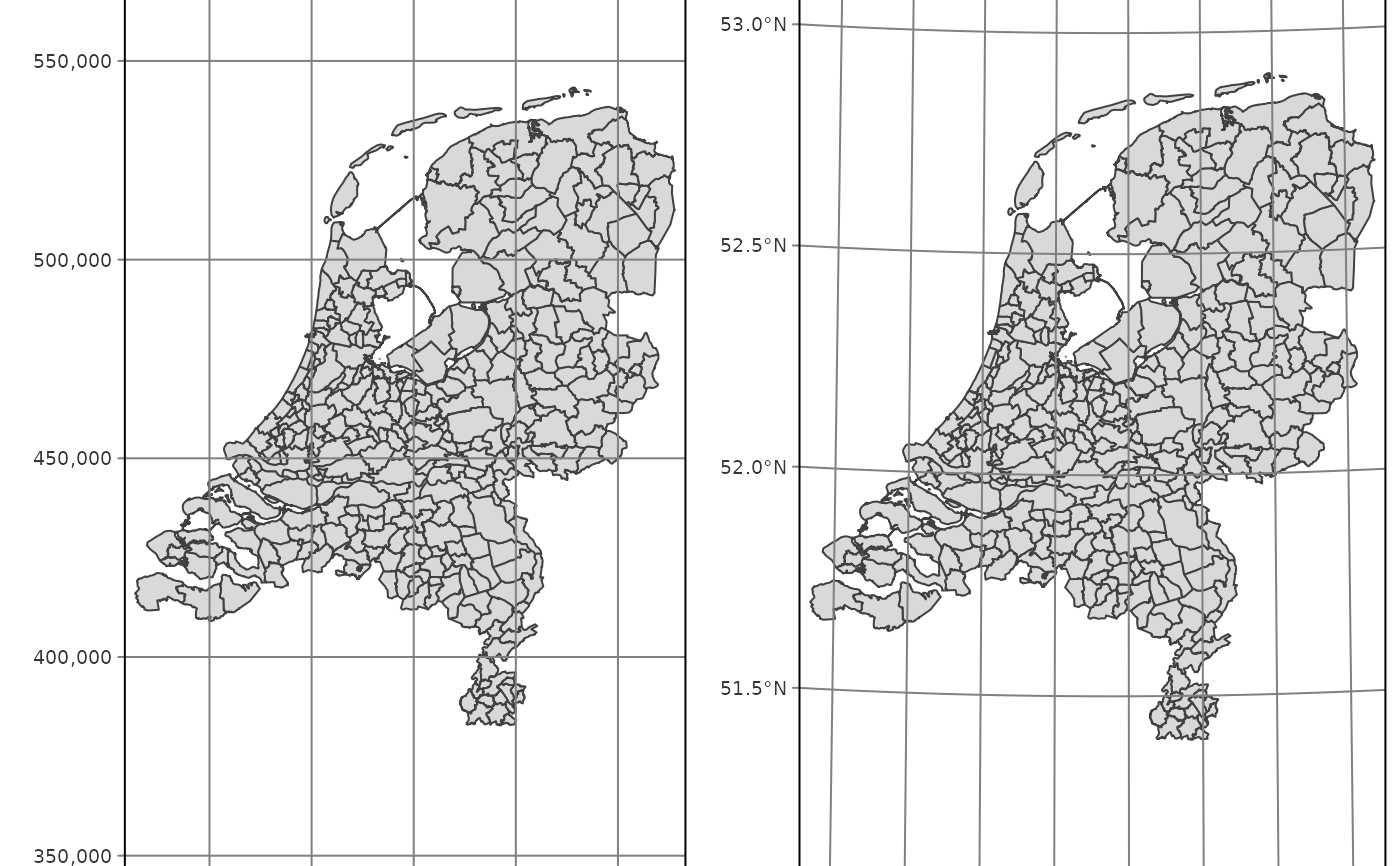qtm(World, shape.crs = "+proj=robin", style = "natural") +
tm_graticules(ticks = FALSE) +
tm_layout(frame=FALSE)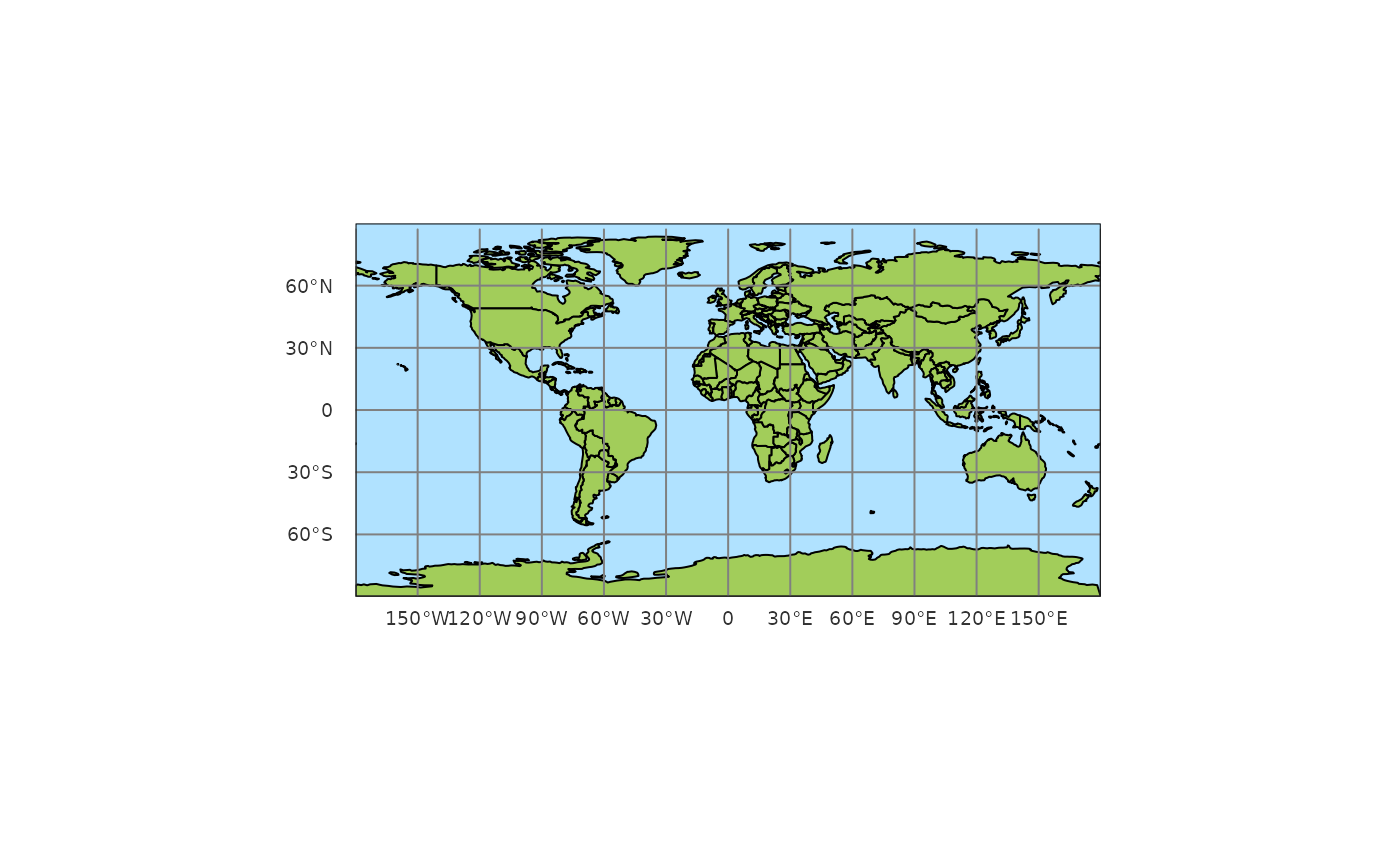tmap_mode(current.mode)
#> tmap mode set to 'plot'